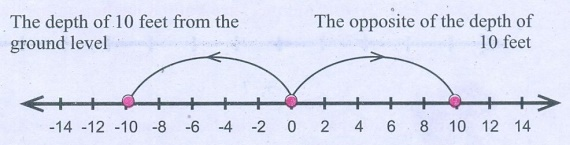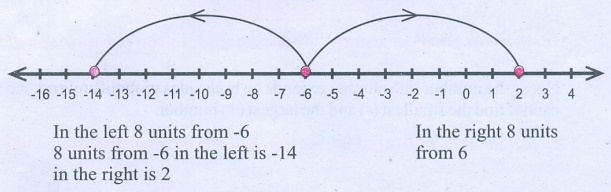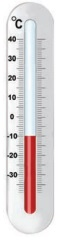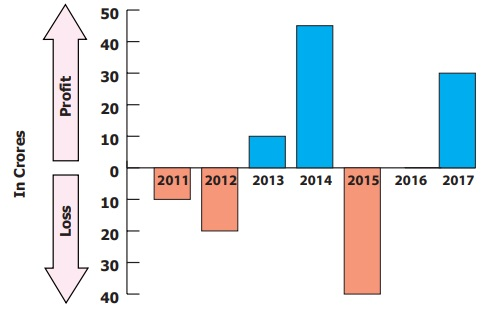Home | | Maths 6th Std | Exercise 2.2

# Exercise 2.2

6th Maths : Term 3 Unit 2 : Integers : Exercise 2.2 : Miscellaneous Practice Problems, Challenge Problems, Text Book Back Exercises Questions with Answers, Solution

Exercise 2.2

Miscellaneous Practice Problems

1. Write two different real life situations that represent the integer −3.

i) a sapling planted at a depth of 3m.

ii) a pit which is 3m deep.

2. Mark the following numbers on a number line

i) All integers which are greater than −7 but less than 7.

ii) The opposite of 3.

iii) 5 units to the left of −1.3. Construct a number line that shows the depth of 10 feet from the ground level and its opposite.The depth of 10 feet from the ground level

The opposite of the depth of 10 feet

4. Identify the integers and mark on the number line that are at a distance of 8 units from −6.

At a distance of 8 units from − 65. Answer the following questions from the number line given below .i) Which integer is greater : G or K ? Why ?

G is left of K. So integer K is greater.

ii) Find the integer that represents C.

−4

iii) How many integers are there between G and H?

6 that is (−2, −1, 0, 1, 2, 3)

iv) Find the pairs of letters which are opposite of a number.

2 pairs they are (C, H) (E, J)

v) Say True or False : 6 units to the left of D is −6.

False. 6 units to the left of D is 0

6. If G is 3 and C is −1, what numbers are A and K on the number line?−3, 7

7. Find the integers that are 4 units to the left 0 and 2 units to the right of −3.

4 units to the left 0 is −4

2 units to the right of −3 is −1

Challenge Problems

8. Is there the smallest and the largest number in the set of integers? Give reason.

No, as the number line number extends on both sides without any end, we cannot find the smallest (−) and the largest (+) number.

9. Look at the Celsius Thermometer and answer the following questions:i) What is the temperature that is shown in the Thermometer?

−10°C

ii) Where will you mark the temperature 5° C below 0° C in the Thermometer?

At − 5°C

iii) What will be the temperature, if 10° C is reduced from the temperature shown in the Thermometer?

− 20°C

iv) Mark the opposite of 15° C in the Thermometer.

−15°C

10. P, Q, R and S are four different integers on a number line. From the following clues, find these integers and write them in ascending order.

i) S is the least of the given integers.

ii) R is the smallest positive integer.

iii) The integers P and S are at the same distance from 0.

iv) Q is 2 units to the left of integer R.

The ascending order is S < Q < O < R < P.

11. Assuming that the home to be the starting point, mark the following places in order on the number line as per instructions given below and write their corresponding integers.Places: Home, School, Library, Playground, Park, Departmental Store, Bus Stand, Railway Station, Post Office, Electricity Board.

Instructions:

i) Bus Stand is 3 units to the right of Home.

ii) Library is 2 units to the left of Home.

iii) Departmental Store is 6 units to the left of Home.

iv) Post Office is 1 unit to the right of the Library.

v) Park is 1 unit right of Departmental Store.

vi) Railway Station is 3 units left of Post Office.

vii) Bus Stand is 8 units to the right of Railway Station.

viii) School is next to the right of Bus Stand.

ix) Playground and Library are opposite to each other.

x) Electricity Board and Departmental Store are at equal distance from Home.i) Bus stand 3

ii) Library – 2

iii) Departmental store −6

iv) Post office – 1

v) Park – 5

vi) Railway Station – 4

vii) Bus stand 3

viii) School 4

ix) Play ground 2

x) Electricity Board 6

12. Complete the table using the following hints:C1: the first non-negative integer.

C3: the opposite to the second negative integer.

C5: the additive identity in whole numbers.

C6: the successor of the integer in C2.

C8: the predecessor of the integer in C7.

C9: the opposite to the integer in C5.13. The following bar graph shows the profit (+) and loss (−) of a small scale company (in crores) between the years 2011 to 2017.i) Write the integer that represents a profit or a loss for the company in 2014?

ii) Denote by an integer on the profit or loss in 2016.

iii) Denote by integers on the loss for the company in 2011 and 2012.

iv) Say True or False: The loss is minimum in 2012.

v) Fill in: The amount of loss in 2011 is equal as profit in 2013.

Exercise 2.2

1. i) a sapling planted at a depth of 3m   ii) a pit which is 3m deep

2.4.5. i) K (–1) ii) −4 iii) 6 (−2, −1, 0, 1, 2, 3) iv) 2 pairs v) False, 0

6. −3, 7

7. −4, −1

8. No, as the number line number extends on both sides without any end, we cannot find the smallest (−) and the largest (+) number

9. i) – 10°C ii) At −5°C iii)−20°C iv) −15°C

10. S<Q<0<R<P

11. i) 3 ii) −2 iii) −6 iv) −1 v) −5 vi) −4 vii) 4 viii) 4  ix) 5 x)2

12. C1: 0, C3: 2, C5: 0, C6: -4, C8: -8, C9: 0

13. i) + 45 ii) 0 iii) −10 & − 20 iv) False v) the same

Tags : Questions with Answers, Solution | Integers | Term 3 Chapter 2 | 6th Maths , 6th Maths : Term 3 Unit 2 : Integers
Study Material, Lecturing Notes, Assignment, Reference, Wiki description explanation, brief detail
6th Maths : Term 3 Unit 2 : Integers : Exercise 2.2 | Questions with Answers, Solution | Integers | Term 3 Chapter 2 | 6th Maths